### Home > PC > Chapter 1 > Lesson 1.3.1 > Problem1-105

1-105.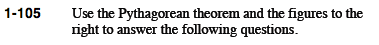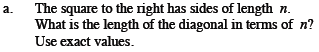Use the Pythagorean Theorem.
n² + n² = d²
Solve for d.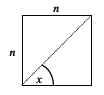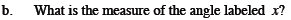What do you know about the interior angles of squares?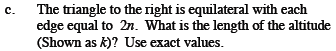The base is cut into two equal parts by k.
Use the Pythagorean Theorem to write an equation and solve for k.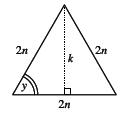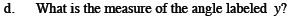What do you know about the interior angles of an equilateral triangle?